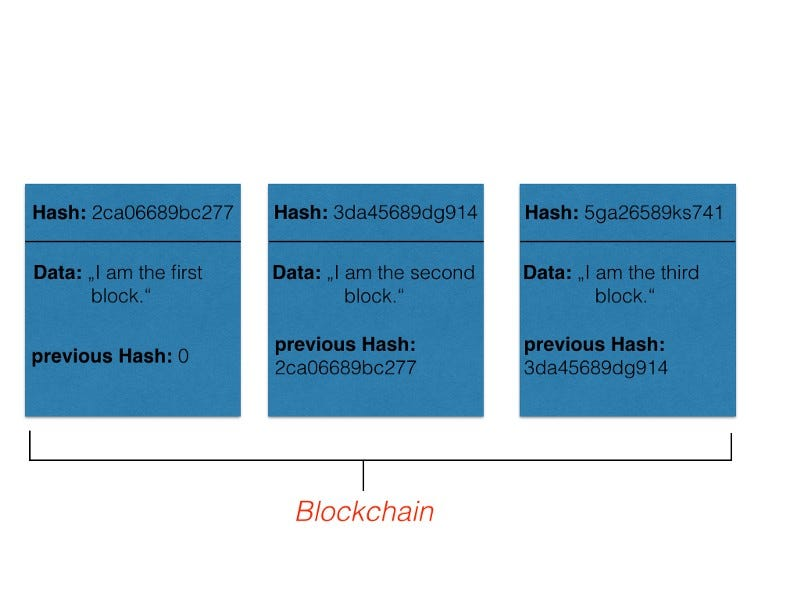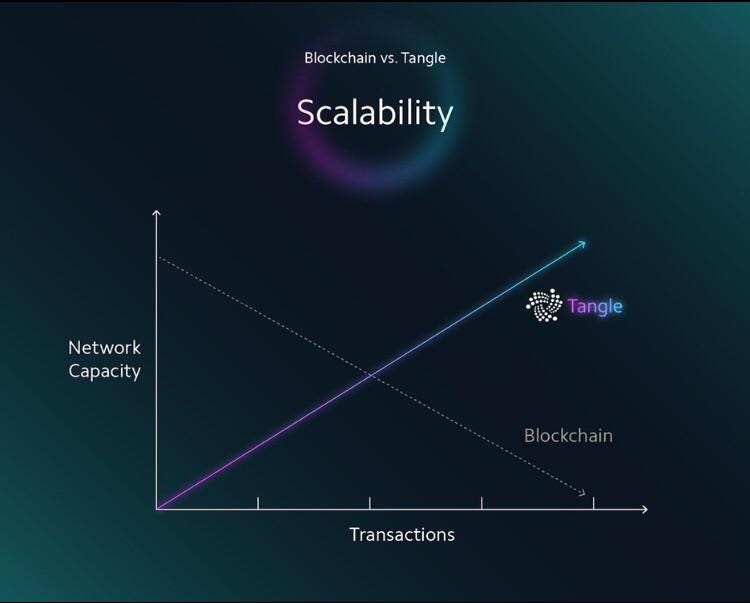Latest Stories

How IOTA solves Blockchains scalability problemBlockchain as a decentralized and cryptographically secured database is one of the new technologies, that will definitely have a huge impact on the future of the digital world. But with an increasing impact comes an increasing number of users — and there a big problem occurs. Most of the currently known blockchain networks are not made for many users — and I mean a lot of users. Let’s just take a look at the most famous blockchain system Bitcoin. The size of a block, which determines the amount of data being able to be stored, is limited to 1MB. As I described in one of my previous articles, How cryptocurrencies actually work, the data stored in cryptocurrency blockchains are transaction data. A block has to be generated and then validated by the network, and this takes time. Thus, there is only a limited amount of transactions, that can be done per a certain amount of time. But since this whole technology gets more and more participants, the time to handle such transactions, increases. This is called the problem of scalability. A solution had to be found, and IOTA did find one.

The IOTA foundation came up with a pretty nice idea — the so called Tangle system. Therefore they used their knowledge from a topic in maths called Graph theory. No worry, I will explain it. First, take a look at a simplified structure of a blockchain in the figure below:We have blocks of information, each block contains certain data and is connected to the previous block via a cryptographically calculated hash value. Now take a look at how the tangle looks like:

What you can see in the figure above, is something that is called a Directed Acyclic Graph in mathematics. For the purpose of understanding it is enough to know, that a graph is just a set of vertices and edges. Directed means, that the edge, that connects two vertices, directs from one vertex to the other. In the tangle system, each transaction is represented by a vertex. That means, if someone is in the process of transferring some IOTA funds, he or she generates a transaction, which then becomes a vertex in the tangle graph above. The clue now is, that every transaction has to approve at least two other transactions. In terms of maths this means, that every vertex has to be connected by directing edges to at least two other vertices. Just look at the figure above and chose some arbitrary vertex — you will see, that there are at least to arrows showing to two other vertices. A transaction is said to be indirectly approved by another transaction, if there is a connection between those two transactions with at least one transaction between them.

So instead of storing transactions in blocks with a limited size, each transaction lives on its own and has to approve other transactions. With this method, the number of transactions, that can be handled in a certain amount of time, increases with the number of transactions! Have a look at the classical scalability figure from the IOTA foundation below.Now that we know, how IOTA solves the scalability problem, it would be pretty interesting to know, how the network of participants is build up.